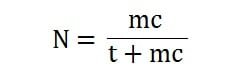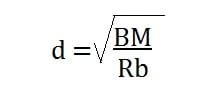# Singly Reinforced Beam Design Procedure

Contents

## Singly Reinforced Beam:

When the area of steel is provided in tension zone only i.e the reinforcement is given only in tension zone, it will be known as singly reinforced beam.

In singly reinforced beam, the reinforcement carries the ultimate bending moment and tension due to bending of the beam. On the other hand, the concrete carries the compression of that beam.

The actual NA of singly reinforced beam is calculated by the below given formula.Generally, these types of beams are balanced, under reinforced or over reinforced type.

In practical work, there is no such way to use reinforcement only in tension area, because we have to bind the stirrups. So, in the compression zone, always two rebars are used to bind the stirrups where, the rebars just withstand those stirrups.

## Singly Reinforced Beam Design Procedure:

1. Determine the value of N by the following formula:[Where N = Critical N.A Constant.]

2. Find the value of J.Where J = Lever arm constant

3. Determine the moment of resistance coefficient4. Select appropriate breadth (b) and equate the bending moment and moment of resistance with the effective depth of the section.5. Calculate the value of AtWhere At = Area of tensile steel.

t = Allowable tensile stress in steel.